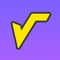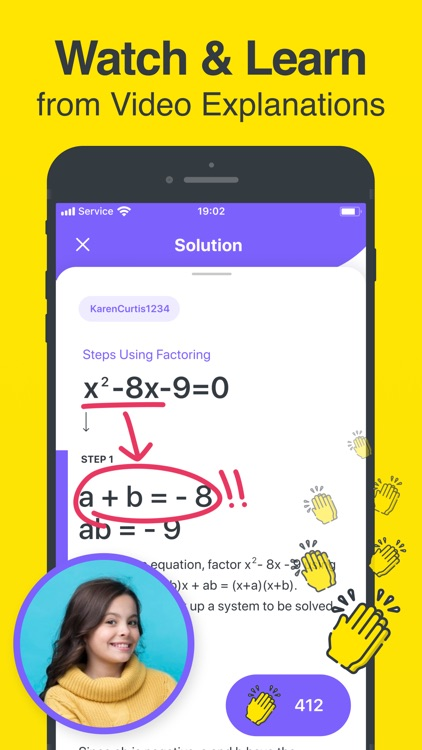## Making Math a Bit Easier# Math Hero: Math Answer Scanner

by PlusMinusOne, Inc.◉ Get unstuck ASAP and learn Math with Math Hero, a FREE step-by-step math solver app!Version
1.4.3
Rating
(1951)
Size
31Mb
Genre
Education
Last updated
October 4, 2021
Release date
May 9, 2021

### App Screenshots### App Store Description

◉ Get unstuck ASAP and learn Math with Math Hero, a FREE step-by-step math solver app!

Got tricky math homework or class assignments? Need instant step-by-step explanations? Tired of waiting for help? Math Hero is here to be your lifesaver.

We've got you covered from simple Arithmetic to advanced Algebra & Calculus. Just scan a math photo, Math Hero instantly recognizes the problem and helps you to solve it with a detailed step-by-step explanation.

With Math Hero, you'll finish your homework faster, prepare better for tests and learn easily with step-by-step explanations so that next time you'll be able to solve the problem yourself.

- KEY FEATURES

◉ Scan printed or handwritten math photo

◉ Get interactive Step-by-Step explanation & Graphing calculator

◉ Scroll through multiple solving methods per problem

◉ Record your notes and explanations for any problem

◉ Watch other's video explanations for problems

◉ Share step by step explanations with your classmates on Snapchat, Whatsapp, iMessage and more

- MATH TOPICS COVERED

◉ Basic Math: arithmetic, integers, decimal numbers, powers, roots, factors

◉ Pre-Algebra: radicals and exponents, fractions, matrices, determinants

◉ Algebra: quadratic equations, system of equations, inequalities, rational expressions, linear, quadratic, and exponential graphs

◉ Calculus: limits, derivatives, integrals, curve sketching

◉ Statistics: Mean, Median, Mode, Standard Deviation, permutations, combinations

ARE YOU THE ONE?

Wait! Are you the one who learns fast and love to share? Then you can be a Math Hero too!

You can use Math Hero to record your own video explanations for any math problem and share with millions of students and be their hero. This way you’ll also learn a lot better and never forget what you learn. Keep in mind, teaching is the best way to learn!